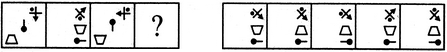Non Verbal Reasoning - Analogy - Discussion

Discussion :: Analogy - Section 1 (Q.No.11)

Each of the following questions consists of two sets of figures. Figures A, B, C and D constitute the Problem Set while figures 1, 2, 3, 4 and 5 constitute the Answer Set. There is a definite relationship between figures A and B. Establish a similar relationship between figures C and D by selecting a suitable figure from the Answer Set that would replace the question mark (?) in fig. (D).

11.

Select a suitable figure from the Answer Figures that would replace the question mark (?).

Problem Figures:                            Answer Figures:(A)     (B)      (C)     (D)                  (1)      (2)      (3)      (4)      (5)

 [A]. 1 [B]. 2 [C]. 3 [D]. 4 [E]. 5

Explanation:

The trapezium gets vertically inverted and move to the middle right position; the pin rotates 90oCW and moves to the lower-right position; the third element rotates 135oACW.

 Akrati said: (Jun 1, 2016) Please explain this again in an easy method.

 Akrati said: (Jun 1, 2016) Please explain this again in an easy method.

 Harry said: (Sep 17, 2017) Can you please explain it deeply?

 Aravinth said: (Mar 17, 2018) Please explain the answer.

 Ganesh Bhattarai said: (Dec 31, 2018) Not getting, please explain the answer detaily.